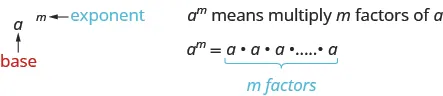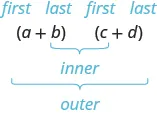Prealgebra

# Key Concepts

PrealgebraKey Concepts

### Key Concepts

#### 10.2Use Multiplication Properties of Exponents

• Exponential NotationThis is read $aa$ to the $mthmth$ power.

• Product Property of Exponents
• If $aa$ is a real number and $m,nm,n$ are counting numbers, then
$am·an=am+nam·an=am+n$
• To multiply with like bases, add the exponents.
• Power Property for Exponents
• If $aa$ is a real number and $m,nm,n$ are counting numbers, then
$(am)n =am⋅n(am)n =am⋅n$
• Product to a Power Property for Exponents
• If $aa$ and $bb$ are real numbers and $mm$ is a whole number, then
$(ab)m=ambm(ab)m=ambm$

#### 10.3Multiply Polynomials

• Use the FOIL method for multiplying two binomials.
 Step 1. Multiply the First terms.Step 2. Multiply the Outer terms. Step 3. Multiply the Inner terms. Step 4. Multiply the Last terms. Step 5. Combine like terms, when possible.
• Multiplying Two Binomials: To multiply binomials, use the:
• Distributive Property
• FOIL Method
• Vertical Method
• Multiplying a Trinomial by a Binomial: To multiply a trinomial by a binomial, use the:
• Distributive Property
• Vertical Method

#### 10.4Divide Monomials

• Equivalent Fractions Property
• If $a,b,ca,b,c$ are whole numbers where $b≠0,c≠0,b≠0,c≠0,$ then
$ab=a·cb·canda·cb·c=abab=a·cb·canda·cb·c=ab$
• Zero Exponent
• If $aa$ is a non-zero number, then $a0=1.a0=1.$
• Any nonzero number raised to the zero power is $1.1.$
• Quotient Property for Exponents
• If $aa$ is a real number, $a≠0,a≠0,$ and $m,nm,n$ are whole numbers, then
$aman=am−n,m>nandaman=1an−m,n>maman=am−n,m>nandaman=1an−m,n>m$
• Quotient to a Power Property for Exponents
• If $aa$ and $bb$ are real numbers, $b≠0,b≠0,$ and $mm$ is a counting number, then
$(ab)m=ambm(ab)m=ambm$
• To raise a fraction to a power, raise the numerator and denominator to that power.

#### 10.5Integer Exponents and Scientific Notation

• Summary of Exponent Properties
• If $a,ba,b$ are real numbers and $m,nm,n$ are integers, then
$Product Propertyam·an=am+nPower Property(am)n=am·nProduct to a Power Property(ab)m=ambmQuotient Propertyaman=am−n,a≠0Zero Exponent Propertya0=1,a≠0Quotient to a Power Property(ab)m=ambm,b≠0Definition of Negative Exponenta−n=1anProduct Propertyam·an=am+nPower Property(am)n=am·nProduct to a Power Property(ab)m=ambmQuotient Propertyaman=am−n,a≠0Zero Exponent Propertya0=1,a≠0Quotient to a Power Property(ab)m=ambm,b≠0Definition of Negative Exponenta−n=1an$
• Convert from Decimal Notation to Scientific Notation: To convert a decimal to scientific notation:
1. Step 1. Move the decimal point so that the first factor is greater than or equal to 1 but less than 10.
2. Step 2. Count the number of decimal places, $nn$, that the decimal point was moved.
3. Step 3.
Write the number as a product with a power of 10.
• If the original number is greater than 1, the power of 10 will be $10n10n$.
• If the original number is between 0 and 1, the power of 10 will be $10n10n$.
4. Step 4. Check.
• Convert Scientific Notation to Decimal Form: To convert scientific notation to decimal form:
1. Step 1. Determine the exponent, $nn$, on the factor 10.
2. Step 2.
Move the decimal $nn$ places, adding zeros if needed.
• If the exponent is positive, move the decimal point $nn$ places to the right.
• If the exponent is negative, move the decimal point $|n||n|$ places to the left.
3. Step 3. Check.

#### 10.6Introduction to Factoring Polynomials

• Find the greatest common factor.
1. Step 1. Factor each coefficient into primes. Write all variables with exponents in expanded form.
2. Step 2. List all factors—matching common factors in a column. In each column, circle the common factors.
3. Step 3. Bring down the common factors that all expressions share.
4. Step 4. Multiply the factors.
• Distributive Property
• If $aa$, $bb$, $cc$ are real numbers, then
$a(b+c)=ab+aca(b+c)=ab+ac$ and $ab+ac=a(b+c)ab+ac=a(b+c)$
• Factor the greatest common factor from a polynomial.
1. Step 1. Find the GCF of all the terms of the polynomial.
2. Step 2. Rewrite each term as a product using the GCF.
3. Step 3. Use the Distributive Property ‘in reverse’ to factor the expression.
4. Step 4. Check by multiplying the factors.
Order a print copy

As an Amazon Associate we earn from qualifying purchases.